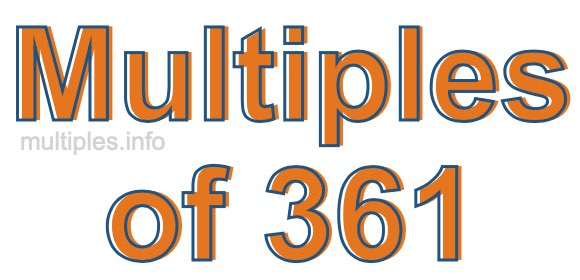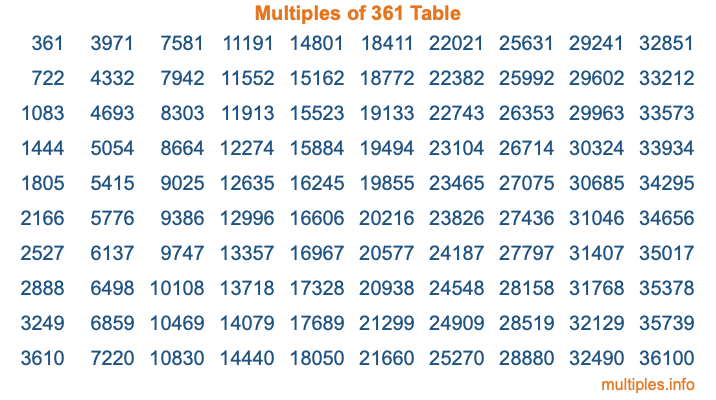Multiples of 361Welcome to the Multiples of 361 page. Here we will first teach you everything you will ever need to know about the multiples of 361, and then give you a study guide summary of everything we taught you to make sure you remember it all. Use this page to look up facts and learn information about the multiples of 361. This page will make you a multiples of three hundred sixty-one expert!

Definition of Multiples of 361
Multiples of 361 are all the numbers that when divided by 361 equal an integer. Each of the multiples of 361 are called a multiple. A multiple of 361 is created by multiplying 361 by an integer.

Therefore, to create a list of multiples of 361, you start with 1 multiplied by 361, then 2 multiplied by 361, then 3 multiplied by 361, and so on for as long as you want. Thus, the list of the first five multiples of 361 is 361, 722, 1083, 1444, and 1805. To see a larger list of multiples of 361, see the printable image of Multiples of 361 further down on this page. We also have a category where you can choose any nth multiple of 361.

Multiples of 361 Checker
The Multiples of 361 Checker below checks to see if any number of your choice is a multiple of 361. In other words, it checks to see if there is any number (integer) that when multiplied by 361 will equal your number. To do that, we divide your number by 361. If the the quotient is an integer, then your number is a multiple of 361.

Is  a multiple of 361?

Least Common Multiple of 361 and ...
A Least Common Multiple (LCM) is the lowest multiple that two or more numbers have in common. This is also called the smallest common multiple or lowest common multiple and is useful to know when you are adding our subtracting fractions. Enter one or more numbers below (361 is already entered) to find the LCM.

Check out our LCM Calculator if you need more details about the Least Common Multiple or if you need the LCM for different numbers for adding and subtraction fractions.

nth Multiple of 361
As we stated above, 361 is the first multiple of 361, 722 is the second multiple of 361, 1083 is the third multiple of 361, and so on. Enter a number below to find the nth multiple of 361.

th multiple of 361

Multiples of 361 vs Factors of 361
361 is a multiple of 361 and a factor of 361, but that is where the similarities end. All postive multiples of 361 are 361 or greater than 361. All positive factors of 361 are 361 or less than 361.

Below is the beginning list of multiples of 361 and the factors of 361 so you can compare:

Multiples of 361: 361, 722, 1083, 1444, 1805, etc.

Factors of 361: 1, 19, 361

As you can see, the multiples of 361 are all the numbers that you can divide by 361 to get a whole number. The factors of 361, on the other hand, are all the whole numbers that you can multiply by another whole number to get 361.

It's also interesting to note that if a number (x) is a factor of 361, then 361 will also be a multiple of that number (x).

Multiples of 361 vs Divisors of 361
The divisors of 361 are all the integers that 361 can be divided by evenly. Below is a list of the divisors of 361.

Divisors of 361: 1, 19, 361

The interesting thing to note here is that if you take any multiple of 361 and divide it by a divisor of 361, you will see that the quotient is an integer.

Multiples of 361 Table
Below is an image of the first 100 multiples of 361 in a table. The table is in chronological order, column by column. The first column has the first ten multiples of 361, the second column has the next ten multiples of 361, and so on.The Multiples of 361 Table is also referred to as the 361 Times Table or Times Table of 361. You are welcome to print out our table for your studies.

Negative Multiples of 361
Although not often discussed or needed in math, it is worth mentioning that you can make a list of negative multiples of 361 by multiplying 361 by -1, then by -2, then by -3, and so on, to get the following list of negative multiples of 361:

-361, -722, -1083, -1444, -1805, etc.

Multiples of 361 Summary
Below is a summary of important Multiples of 361 facts that we have discussed on this page. To retain the knowledge on this page, we recommend that you read through the summary and explain to yourself or a study partner why they hold true.

There are an infinite number of multiples of 361.

A multiple of 361 divided by 361 will equal a whole number.

361 divided by a factor of 361 equals a divisor of 361.

The nth multiple of 361 is n times 361.

The largest factor of 361 is equal to the first positive multiple of 361.

361 is a multiple of every factor of 361.

361 is a multiple of 361.

A multiple of 361 divided by a divisor of 361 equals an integer.

361 divided by a divisor of 361 equals a factor of 361.

Any integer times 361 will equal a multiple of 361.

Multiples of a Number
Here you can get the multiples of another number, all with the same attention to detail as we did for multiples of 361 on this page.

Multiples of
Multiples of 362
Did you find our page about multiples of three hundred sixty-one educational? Do you want more knowledge? Check out the multiples of the next number on our list!

Copyright  |   Privacy Policy  |   Disclaimer  |   Contact